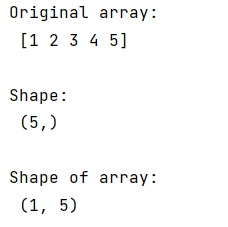# How to fix 'Why does the shape of a 1D array not show the number of rows as 1'?

Learn about the shape() function and why does shape() function does not given the number of rows as 1 for a 1D array?
Submitted by Pranit Sharma, on March 10, 2023

## Python - numpy.shape() Method

The numpy.shape(a) is used to return the shape of a numpy array (a). By this, we mean that this function gives the dimensions of a numpy array i.e., the number of rows and columns.

It returns a tuple with two values, the first is the number of rows and the other is the number of columns.

If we apply numpy.shape() on a 1D numpy array, it will not return the number of rows as 1. This is because the concept of rows and columns applies when you have a 2D array. However, if we want the shape function to return 1 as the number of rows, we can convert it into a 2D array by giving only one row.

Let us understand with the help of an example,

## Python program to demonstrate the example of numpy.shape()

```# Import numpy
import numpy as np

# Creating a numpy array
arr = np.array([1,2,3,4,5])

# Display original array
print("Original array:\n",arr,"\n")

# Display shape
print("Shape:\n",arr.shape,"\n")

# Converting into 2d array and returning shape
arr = np.array([[1,2,3,4,5]])
print("Shape of array:\n",arr.shape,"\n")
```

### OutputLanguages: » C » C++ » C++ STL » Java » Data Structure » C#.Net » Android » Kotlin » SQL
Web Technologies: » PHP » Python » JavaScript » CSS » Ajax » Node.js » Web programming/HTML
Solved programs: » C » C++ » DS » Java » C#
Aptitude que. & ans.: » C » C++ » Java » DBMS
Interview que. & ans.: » C » Embedded C » Java » SEO » HR
CS Subjects: » CS Basics » O.S. » Networks » DBMS » Embedded Systems » Cloud Computing
» Machine learning » CS Organizations » Linux » DOS
More: » Articles » Puzzles » News/Updates